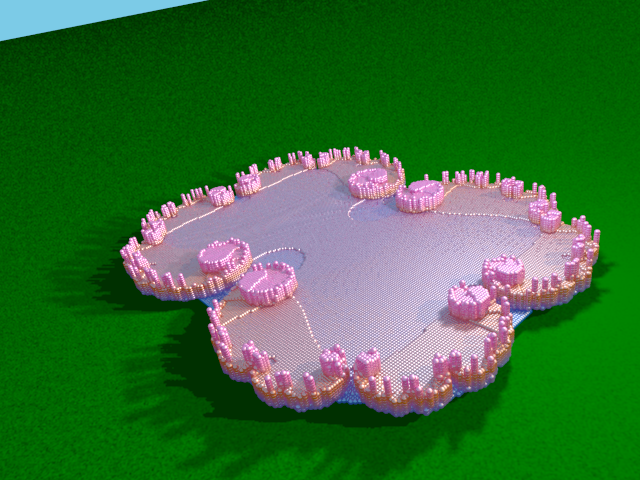# Order from Order: 3D Fractal Landscapes

Fractal Landscapes plotted as wholes (mesh method)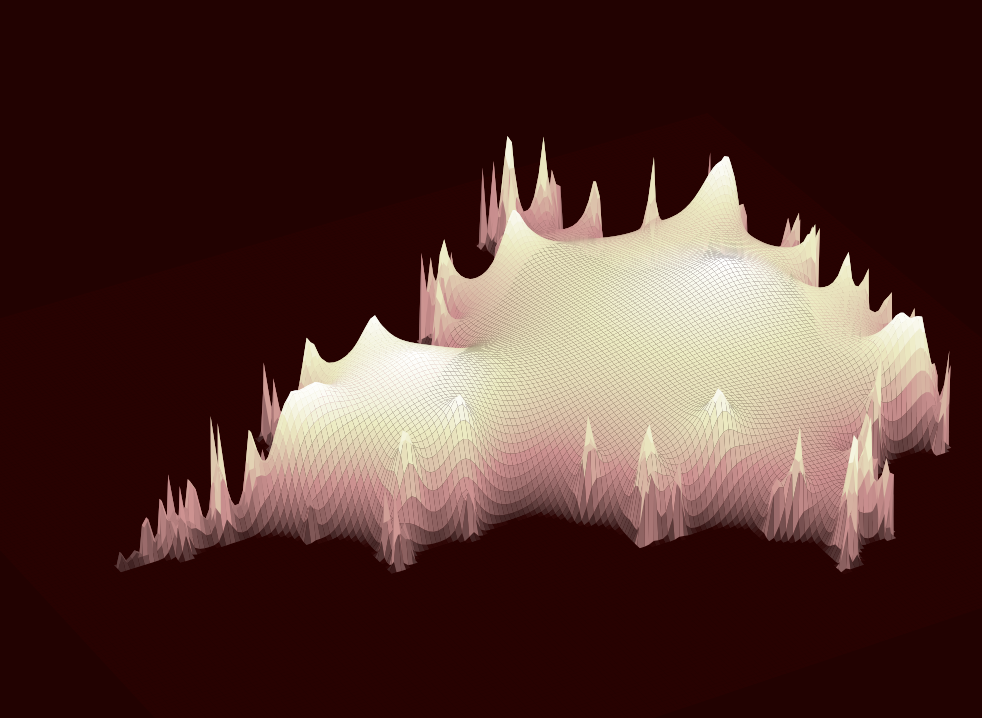Mandelbrot hill (mesh plot)

Python 3 codeJulia hill (mesh plot)

Python 3 code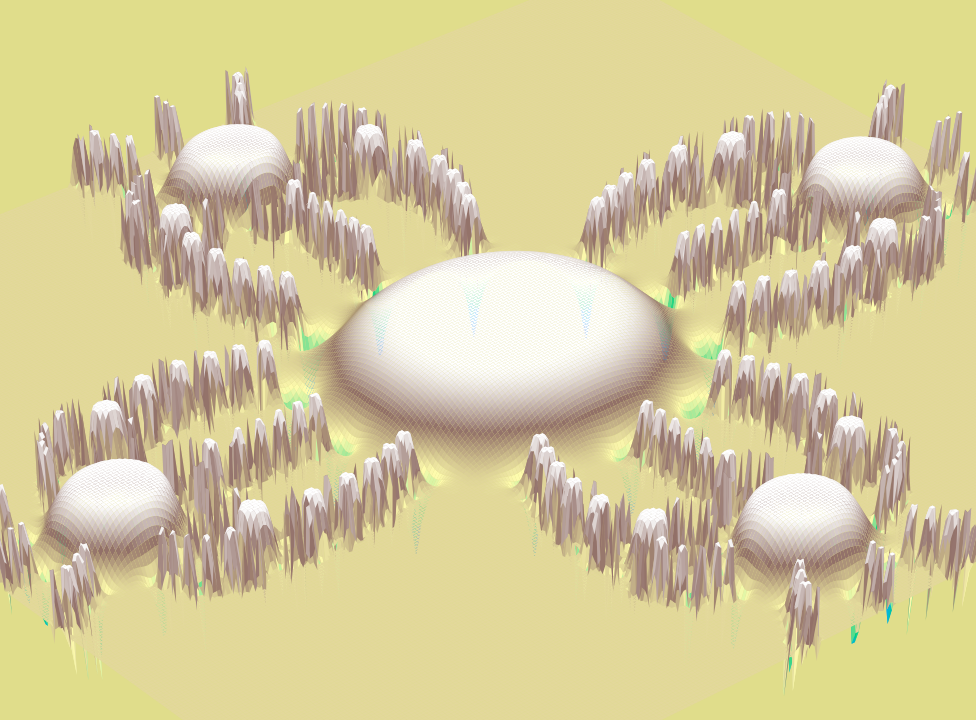Newton method hill (mesh plot)

Python 3 codeMandelbrot valley (mesh plot)

Python 3 code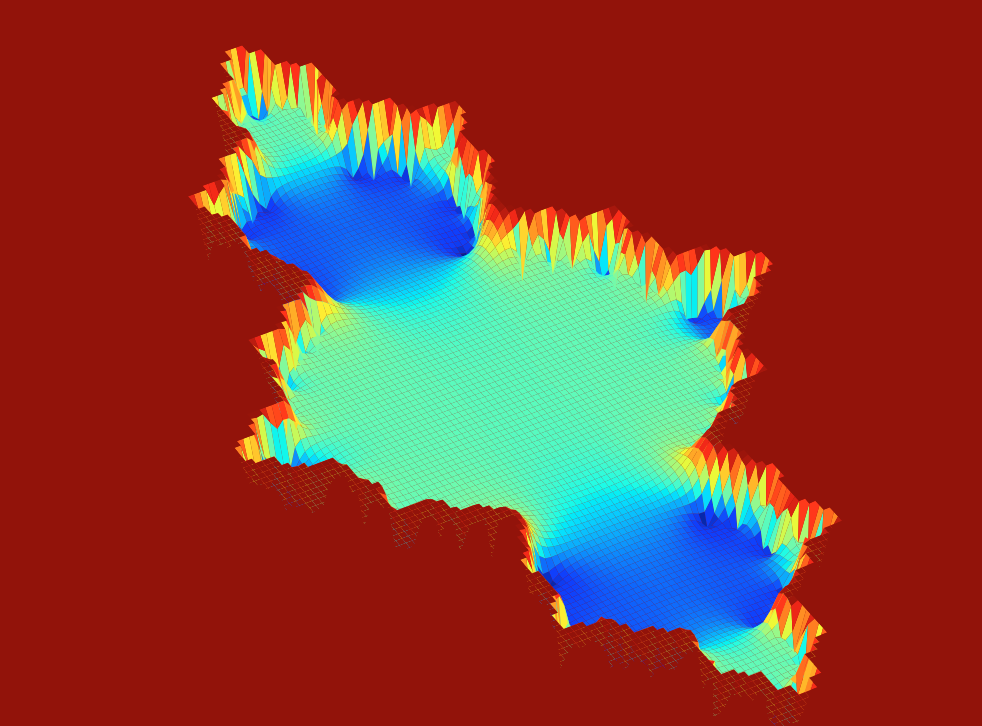Julia valley (mesh plot)

Python 3 codeNewton method valley (mesh plot)

Python 3 code

Fractal Landscapes plotted as wholes (contour method)Mandelbrot hill (contour plot)

Python 3 code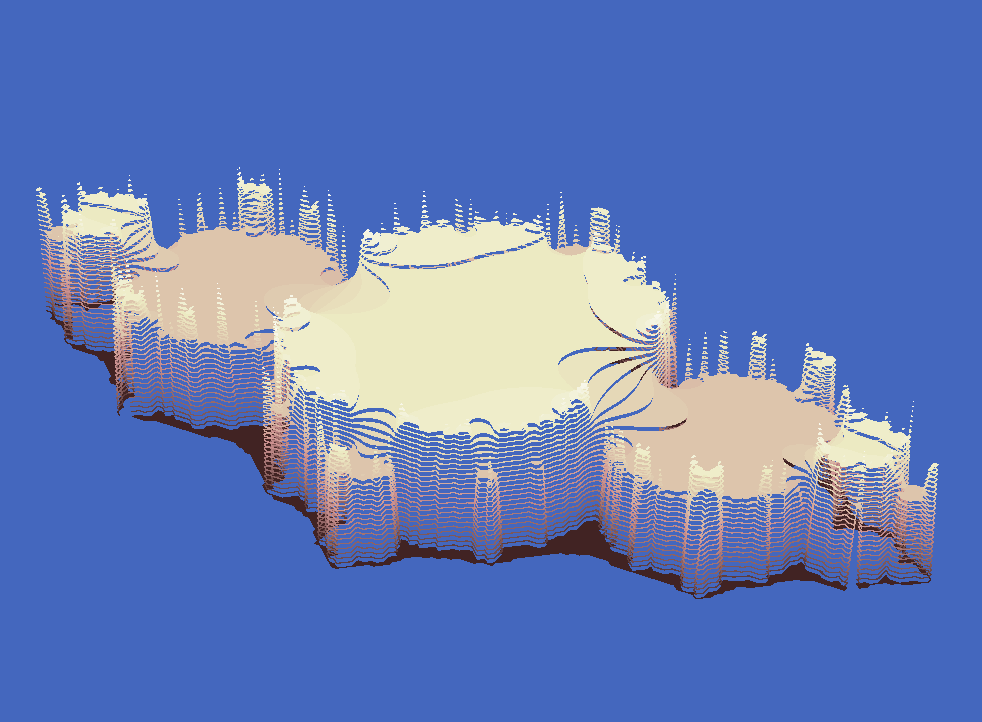Julia hill (contour plot)

Python 3 codeNewton method hill (contour plot)

Python 3 code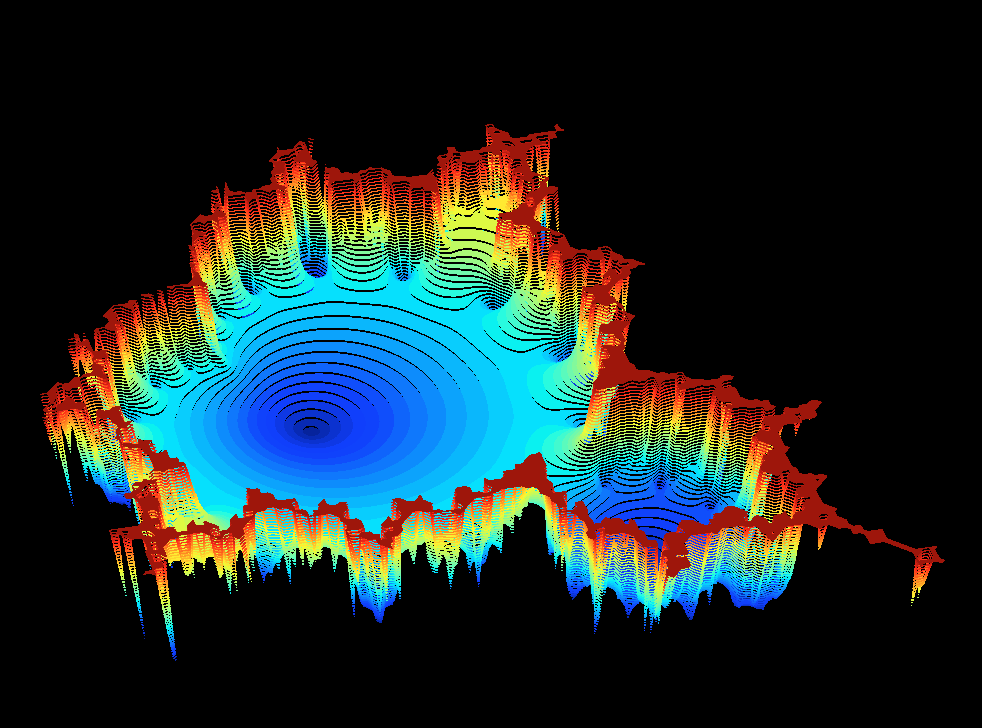Mandelbrot valley (contour plot)

Python 3 codeJulia valley (contour plot)

Python 3 code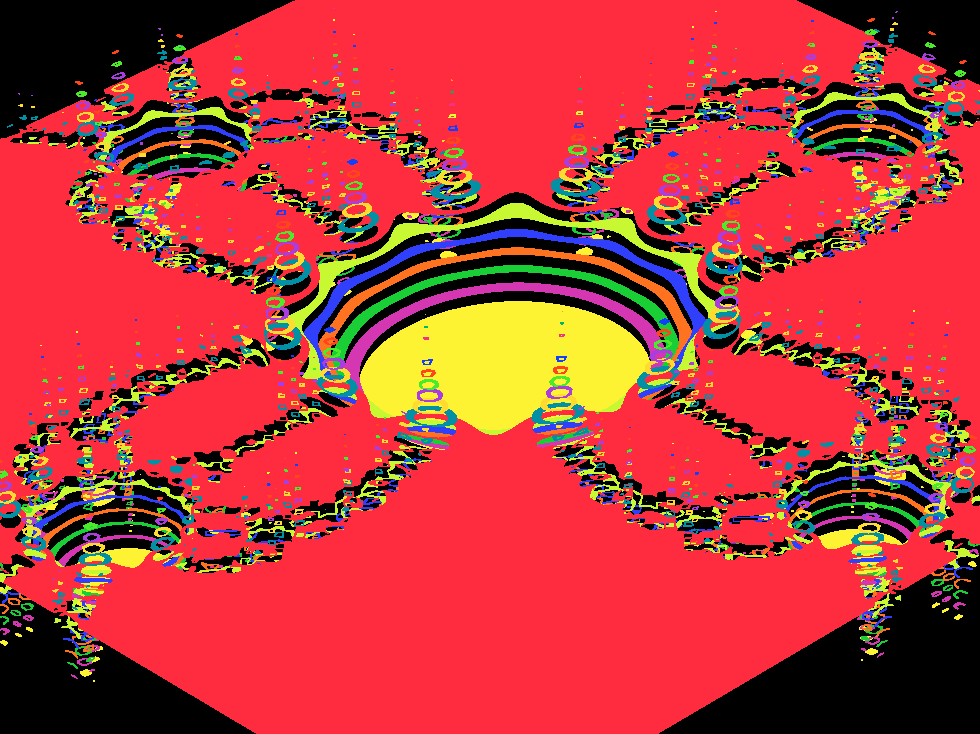Newton method valley (contour plot)

Python 3 code

Fractal Landscapes plotted sequentiallyMandelbrot set (z^2+c, zo=0)

POV Ray 3.7 code

View animation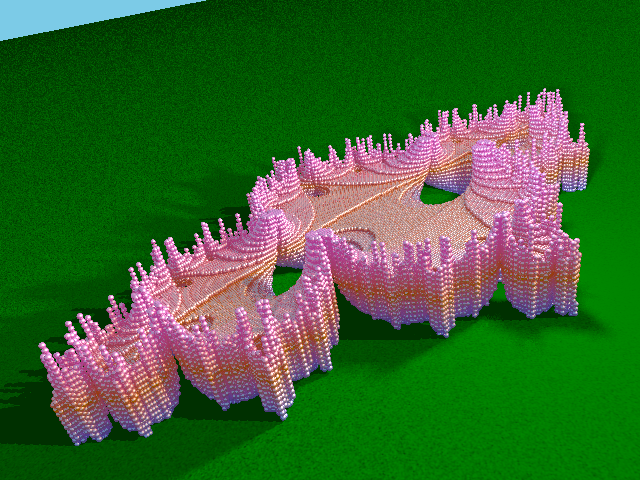Julia set (z^2+c, c=−0.7454294)

POV Ray 3.7 code

View animation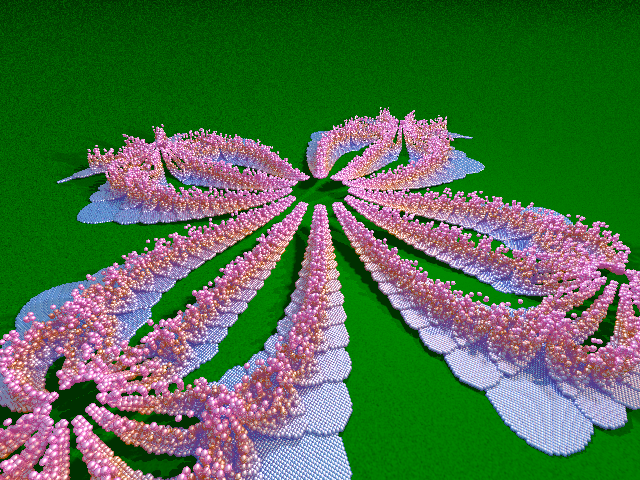Newton method set (z^4+1=0)

POV Ray 3.7 code

View animation

step 1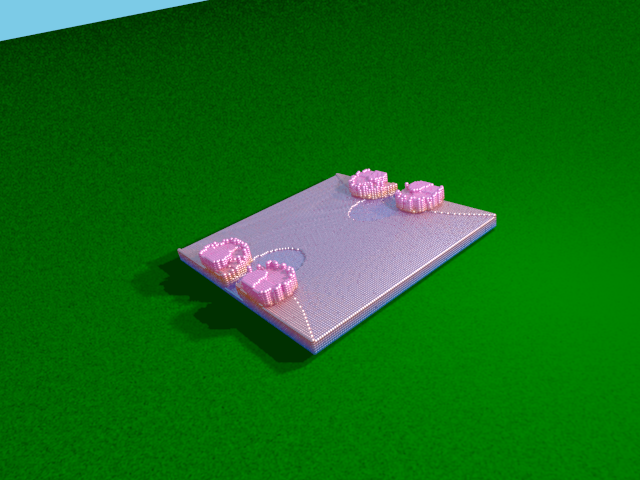step 2Julia set (z^2+c, c=0.27334)

POV Ray 3.7 code

View animation

step 3